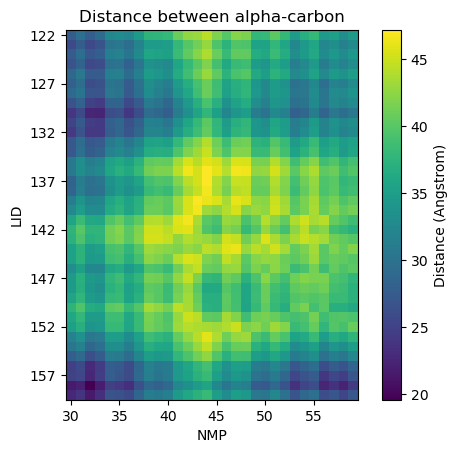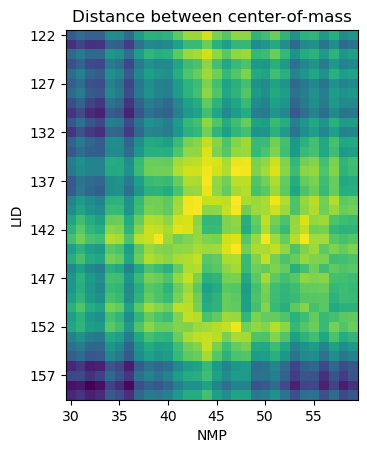# All distances between two selections

Here we use distances.distance_array to quantify the distances between each atom of a target set to each atom in a reference set, and show how we can extend that to calculating the distances between the centers-of-mass of residues.

Last updated: December 2022 with MDAnalysis 2.4.0-dev0

Minimum version of MDAnalysis: 0.19.0

Packages required:

Optional packages for visualisation:

:

import MDAnalysis as mda
from MDAnalysis.tests.datafiles import PDB_small
from MDAnalysis.analysis import distances

import numpy as np
import matplotlib.pyplot as plt
%matplotlib inline

import warnings
# suppress some MDAnalysis warnings when writing PDB files
warnings.filterwarnings('ignore')


The test files we will be working with here feature adenylate kinase (AdK), a phosophotransferase enzyme. ([BDPW09]) AdK has three domains:

• CORE

• LID: an ATP-binding domain (residues 122-159)

• NMP: an AMP-binding domain (residues 30-59)

:

u = mda.Universe(PDB_small)   # open AdK


## Calculating atom-to-atom distances between non-matching coordinate arrays

We select the alpha-carbon atoms of each domain.

:

LID_ca = u.select_atoms('name CA and resid 122-159')
NMP_ca = u.select_atoms('name CA and resid 30-59')

n_LID = len(LID_ca)
n_NMP = len(NMP_ca)
print('LID has {} residues and NMP has {} residues'.format(n_LID, n_NMP))

LID has 38 residues and NMP has 30 residues


distances.distance_array(API docs) will produce an array with shape (n, m) distances if there are n positions in the reference array and m positions in the other configuration. If you want to calculate distances following the minimum image convention, you must pass the universe dimensions into the box keyword.

:

dist_arr = distances.distance_array(LID_ca.positions, # reference
NMP_ca.positions, # configuration
box=u.dimensions)
dist_arr.shape

:

(38, 30)


### Plotting distance as a heatmap

:

fig, ax = plt.subplots()
im = ax.imshow(dist_arr, origin='upper')

# add residue ID labels to axes
tick_interval = 5
ax.set_yticks(np.arange(n_LID)[::tick_interval])
ax.set_xticks(np.arange(n_NMP)[::tick_interval])
ax.set_yticklabels(LID_ca.resids[::tick_interval])
ax.set_xticklabels(NMP_ca.resids[::tick_interval])

# add figure labels and titles
plt.ylabel('LID')
plt.xlabel('NMP')
plt.title('Distance between alpha-carbon')

# colorbar
cbar = fig.colorbar(im)
cbar.ax.set_ylabel('Distance (Angstrom)')

:

Text(0, 0.5, 'Distance (Angstrom)')## Calculating residue-to-residue distances

As distances.distance_array just takes coordinate arrays as input, it is very flexible in calculating distances between each atom, or centers-of-masses, centers-of-geometries, and so on.

Instead of calculating the distance between the alpha-carbon of each residue, we could look at the distances between the centers-of-mass instead. The process is very similar to the atom-wise distances above, but we give distances.distance_array an array of residue center-of-mass coordinates instead.

:

LID = u.select_atoms('resid 122-159')
NMP = u.select_atoms('resid 30-59')

LID_com = LID.center_of_mass(compound='residues')
NMP_com = NMP.center_of_mass(compound='residues')

n_LID = len(LID_com)
n_NMP = len(NMP_com)

print('LID has {} residues and NMP has {} residues'.format(n_LID, n_NMP))

LID has 38 residues and NMP has 30 residues


We can pass these center-of-mass arrays directly into distances.distance_array.

:

res_dist = distances.distance_array(LID_com, NMP_com,
box=u.dimensions)


### Plotting

:

fig2, ax2 = plt.subplots()
im2 = ax2.imshow(res_dist, origin='upper')

# add residue ID labels to axes
tick_interval = 5
ax2.set_yticks(np.arange(n_LID)[::tick_interval])
ax2.set_xticks(np.arange(n_NMP)[::tick_interval])
ax2.set_yticklabels(LID.residues.resids[::tick_interval])
ax2.set_xticklabels(NMP.residues.resids[::tick_interval])

# add figure labels and titles
plt.ylabel('LID')
plt.xlabel('NMP')
plt.title('Distance between center-of-mass')

# colorbar
cbar2 = fig2.colorbar(im)
cbar2.ax.set_ylabel('Distance (Angstrom)')

:

Text(0, 0.5, 'Distance (Angstrom)')